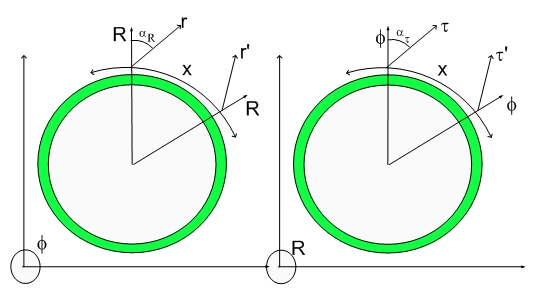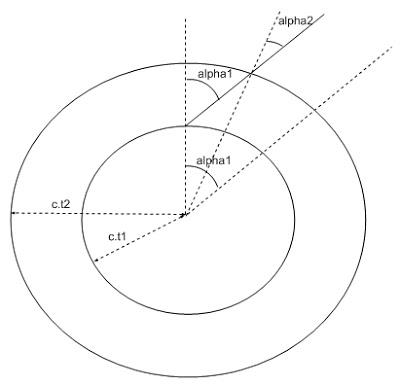# Newton's First Law...Newton's First Law

I mentioned that I was afraid that my legacy would be my Balls Diagram...:) but I am afraid I will have to challenge Newton's Laws also and them only my Intellectual Courage or Audacity will be remembered...:)

First let's review Newton's Laws with the help of Wikipedia:

The Three Laws of Motion
Newton's Laws of Motion describe the acceleration of massive objects. The modern understanding of Newton's three laws of motion is:

First Law
If no external force acts on a particle, then it is possible to select a set of reference frames observed from which the particle is seen to move without any change in its velocity. These reference frames are called inertial reference frames.

Second Law
When observed from an inertial reference frame, the vector sum of all forces on a particle is proportional to the rate of change of its linear momentum with respect to time. Momentum is the product of mass and velocity. (In special relativity, a third factor is included — the Lorentz factor.) Notice that this is only true when the observation is made from an inertial reference frame, and since an inertial reference frame is defined by the first law, asking a proof of the first law from the second law is a fallacy.

Third Law
Whenever A exerts a force on B, B simultaneously exerts a force on A with the same magnitude in the opposite direction. The strong form of the law further postulates that these two forces act along the same line.

The first Law was tackled in my paper where I provided a simple and clear explanation why things that are moving will keep moving at the same speed if there are no forces acting upon them. Of course, since my theory is geometrical in nature, that explanation will have to have space, space deformation and space relaxation. I will replay the argument here in a slightly syncopated fashion due the juxtaposition of the three laws and my effort to address each on its time..:)

Here is the plot that explains why Newton's first law exists:At time t1 the 4D radius of the Universe was c.t1. Let's say that a force was momentarily applied to a body (initially at rest with the Fabric of Space) and it shots off at angle alpha1. It is clear that in this R versus X cross section of the Universe that the angle alpha1 is given by artan (v/c). It is also clear that after some time, at time t2 the angle alpha2 with the perpendicular to the Fabric of Space (relaxed 3D hypersurface) is smaller due to relaxation/motion.

I proposed that this relaxation process is the reason for motion in my paper and replicate the argument here for your enjoyment.

This takes care of the first law. The second law has to do with Inertia.

I will tackle the Newton's Second Law tomorrow. The clue is that when you see a law your have to question it..:) Be a rebel... think...:) There are so many physical questions behind the simplest mathematical equation....

Cheers,

MP

Currently unrated

### New Comment

required

required (not published)

optional

required